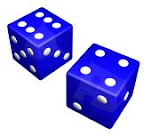## Sunday, August 31, 2008

### Factoring dice

Required reading for this post: Generating functionsWe have two normal six-sided dice. We roll both of them, and sum the number of pips. There is a certain probability that we get a 2, or a 3, or a 4, and so on all the way to 12. What we want is a new pair of six-sided dice with the exact same probabilities as the normal dice, but with different numbers of pips on the faces. Each side must have a positive integer number of pips. Can you create such a pair of dice?
To solve this problem, we must have a better understanding of generating functions. Each sequence generates a unique function. Each function corresponds to a unique sequence. If we add two generating functions together, the resulting function corresponds to the sum of their respective sequences. But what happens if we multiply generating functions together?

Let's say we have some arbitrary sequence {a0, a1, a2, a3, a4, a5, ...} and another arbitrary sequence {b0, b1, b2, b3, b4, b5, ...}. Let's see what happens when we multiply them together.
F = a0 + a1*x + a2*x2 + a3*x3 + a4*x4 + a5*x5 + ...
G = b0 + b1*x + b2*x2 + b3*x3 + b4*x4 + b5*x5 + ...
F*G = a0*b0 + (a0*b1 + a1*b0)*x + (a0*b2 + a1*b1 + a2*b0)*x2 + ...
It might be a little difficult to make sense out of what's going on here. It's important to pay attention to the subscripts. Pay attention to the sum of the subscripts. I'll make this a bit more explicit.All the sums in the first box add up to zero. All the sums in the next box add up to 1. The sums in the next box add up to 2. Note that every possible way sum to 2 with two non-negative integers is represented in that box. This pattern will continue. The next box would contain every possible way to sum to 3. The next box contains all the sums to 4. And so on.

Have you figured out yet how this might relate to the dice? The answer follows.

We can say that each sequence represents some sort of generalized dice. aj represents the number of ways for dice "a" to roll the number j, and bk represents the number of ways for dice "b" to roll the number k. If we multiply the functions generated by each sequence, then we get a new sequence {c0, c1, c2, c3, c4, c5, ...}. cn represents the number of ways for dice "a" and "b" to have a sum of n.

We can prove that this is the case. If we take any term cn, it can be represented by the following sum:

cn = an*b0 + an-1*b1 + ... + a1*bn-1 + a0*bn

If we recall the definition of aj and bk, the proof becomes obvious. The first term "an*b0" represents the number of ways to roll n on dice "a" and 0 on dice "b". The next term represents the number of ways to roll n-1 on dice "a" and 1 on dice "b". And so on.

So let's try it with normal six-sided dice!

We can easily get the generating function D for a normal six-sided dice, and the generating function D*D for two normal dice.
Sequence for normal dice: {0, 1, 1, 1, 1, 1, 1, 0, 0, 0, ...}
Let D = generating function for normal dice
D = x + x2 + x3 + x4 + x5 + x6
D = x * (1 + x) * (1 + x2 + x4)
D = x * (1 + x) * (1 + x + x2) * (1 - x + x2)
D*D = x2 * (1 + x)2 * (1 + x + x2)2 * (1 - x + x2)2
Now what we want are two new six-sided dice with generating functions A and B such that A*B = D*D. So what we have to do is split D*D into two groups of factors, one for A and one for B. Each group must have the factor x, because that ensures that each side has a nonzero number of pips. Each group must also have (1 + x) * (1 + x + x2), to ensure that they have exactly six sides. However, we can split the factor (1 - x + x2)2 in any way we want.
A = x * (1 + x) * (1 + x + x2) * (1 - x + x2)2
B = x * (1 + x) * (1 + x + x2)
A = x + x3 + x4 + x5 + x6 + x8
B = x + 2x2 + 2x3 + x4
These correspond to dice with sides {1, 3, 4, 5, 6, 8} and {1, 2, 2, 3, 3, 4}. There's your solution, right there.

Well, that was quite a mathematical adventure! I've been having fun factoring other kinds of dice as well.

#### 2 comments:

Barry Leiba said...

This post has been included in the 40th Carnival of Mathematics. Come check out the others.Anonymous said...

This is fun. I teach high school kids dice problems. And my text covers this, but honestly... Your stuff is too good, which means I have to read up on generating functions...

Thanks